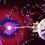# Reaction between Sulphur and Oxygen

I had been asked by one of my chemistry teachers to find out about an equation. The equation is:

$$3S + 4O_{2} \to SO_{2} + 2SO_{3}$$

$4S + 5O_{2} \to 2SO_{2} + 2SO_{3}$

How is it possible than an equation can be balanced in two ways even when it is in the simplest form ?

Also this equation cannot be balanced by P.O.A.C. method.

Please anyone help me out and enlighten me on this topic.Note by Rishabh Tripathi
6 years, 3 months ago

This discussion board is a place to discuss our Daily Challenges and the math and science related to those challenges. Explanations are more than just a solution — they should explain the steps and thinking strategies that you used to obtain the solution. Comments should further the discussion of math and science.

When posting on Brilliant:

• Use the emojis to react to an explanation, whether you're congratulating a job well done , or just really confused .
• Ask specific questions about the challenge or the steps in somebody's explanation. Well-posed questions can add a lot to the discussion, but posting "I don't understand!" doesn't help anyone.
• Try to contribute something new to the discussion, whether it is an extension, generalization or other idea related to the challenge.

MarkdownAppears as
*italics* or _italics_ italics
**bold** or __bold__ bold
- bulleted- list
• bulleted
• list
1. numbered2. list
1. numbered
2. list
Note: you must add a full line of space before and after lists for them to show up correctly
paragraph 1paragraph 2

paragraph 1

paragraph 2

[example link](https://brilliant.org)example link
> This is a quote
This is a quote
    # I indented these lines
# 4 spaces, and now they show
# up as a code block.

print "hello world"
# I indented these lines
# 4 spaces, and now they show
# up as a code block.

print "hello world"
MathAppears as
Remember to wrap math in $$ ... $$ or $ ... $ to ensure proper formatting.
2 \times 3 $2 \times 3$
2^{34} $2^{34}$
a_{i-1} $a_{i-1}$
\frac{2}{3} $\frac{2}{3}$
\sqrt{2} $\sqrt{2}$
\sum_{i=1}^3 $\sum_{i=1}^3$
\sin \theta $\sin \theta$
\boxed{123} $\boxed{123}$

Sort by:

Sulphur is going into different oxidation states in sulphur dioxide and sulphur trioxide. So in the first equation one of the sulphur atoms is getting oxidised into sulphur dioxide and the other two into sulphur trioxide. In the second one two sulphur atoms each are going into sulphur dioxide and troxide. Any such reaction could be made with some $x$ sulphur atoms getting oxidised into dioxide and $y$ into trioxide. Now the question is how is this possible that different number of sulphur atoms are getting oxidised in the reactions. The answer is simple. It is because the above reaction is actually a combination of two reactions - one being $S + O_2 \rightarrow SO_2$ and the other being $2 S + 3 O_2 \rightarrow 2 SO_3$. So if the first reaction is added two the second one you would obtain the first reaction given by your teacher. Figure out what happens when you add $2$ times the first reaction to the second reaction.

Realize the idea?

Hope this helps.

- 6 years, 3 months ago

Nice explanation.

- 6 years, 3 months ago

Thanks.

- 6 years, 3 months ago

yeah.. after doing it gives another balanced equation that too in simplest form. So, this reaction as a whole cannot be possible without breaking it into two different reactions ?

- 6 years, 3 months ago

The reaction is actually a linear combination of two reactions. The question isn't whether the reaction is possible by breaking it into two different reactions.

- 6 years, 3 months ago

I see. Well Thank you.. :)

- 6 years, 3 months ago

Mathematically, this can be explained as follows. Suppose that you can balance the equation as follows.

$aS+bO_2\rightarrow cSO_2+dSO_3$

Now, comparing the number of sulfur and oxygen atoms on both sides,

$a=c+d$ and $2b=2c+3d$

Now suppose you want $a,b,c,d$ to be integers. It is clear from the second equation that $d$ must be even. Hence $d=2D$. So $(a,b,c,d)=(c+2D,c+3D,c,2D)$ for any pair of positive integers $c$ and $D$. In your first reaction you have $(c,D)=(1,1)$ and in the second reaction, you have $(c,D)=(2,1)$. You can find other solutions for other values of $c$ and $d$.

I don't know if there is a chemical reason behind this.

- 6 years, 3 months ago

Your explanation doesn't make it clear why this actually happens.

- 6 years, 3 months ago

Well, I find Sudeep Salgia's answer to be more insightful, especially because he divides the equation into two separate equations. My answer basically shows you how to convert this into a number theoretic problem.

- 6 years, 3 months ago

IMO, You explained it better.

- 6 years, 3 months ago

IMO ?? What it means here ?

- 6 years, 3 months ago

maybe IMO mean international mathematical olympaid...anyway... i do not sure about possibilities comming with extra bonding in the sulphur atomic model or sulphur molecule.

- 4 years, 10 months ago